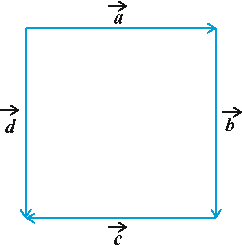“It’s not what you look at that matters, it’s what you see.” –Anonymous

Topics discussed in this video:

Physical Quantity, Scalar and Vector Quantity, Graphical representation of vectors, Algebraical representation of vectors, Parallel Vectors, Co-linear Vectors, Opposite Vectors, Equal Vectors and Position Vectors.

 NCERT EXERCISE 10.1

1. Represent graphically a displacement of 40 km, 30° east of north.

2. Classify the following measures as scalars and vectors.
(i) 10 kg
(ii) 2 meters north-west
(iii) 40°
(iv) 40 watt
(v) 10-19 coulomb
(vi) 20 m/s2

3. Classify the following as scalar and vector quantities.

(i) time period
(ii) distance
(iii) force
(iv) velocity
(v) work done

4. In Fig 10.6 (a square), identify the following vectors.
(i) Coinitial
(ii) Equal
(iii) Collinear but not equal5. Answer the following as true or false.
(i) a and –a are collinear.
(ii) Two collinear vectors are always equal in magnitude.
(iii) Two vectors having same magnitude are collinear.
(iv) Two collinear vectors having the same magnitude are equal.